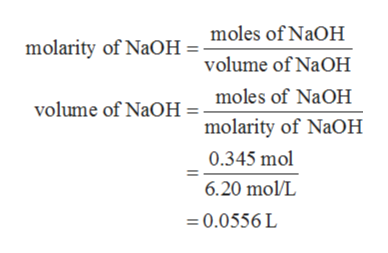# How many milliliters of a 6.20 M NaOH solution are needed to provide 0.345 mol of NaOH?

Question
48 views

How many milliliters of a 6.20 M NaOH solution are needed to provide 0.345 mol of NaOH?

check_circle

Step 1

The values are given as follows:

Step 2

The required amount of volume of NaOH can be calculated with the help of molarity formula as follows:help_outlineImage Transcriptionclosemolarity of NaOH = moles of NaOH volume of NaOH moles of NaOH volume of NaOH molarity of NaOH 0.345 mol 6.20 mol/L 0.0556 L fullscreen
Step 3

The volume of NaOH is converted i...

### Want to see the full answer?

See Solution

#### Want to see this answer and more?

Solutions are written by subject experts who are available 24/7. Questions are typically answered within 1 hour.*

See Solution
*Response times may vary by subject and question.
Tagged in

### Chemistry